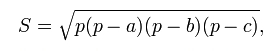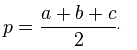# Calculating area of a triangle using the formula of Heron

The calculator below makes the calculation area of a triangle on the well-known formula of Heron.

Here is the formula itself:where p - semiperimeter triangle:If anyone is interested to learn more about Heron's formula, then you can easily do it on the page:

https://en.wikipedia.org/wiki/Heron's_formula

﻿

Calculation square triangle at the formula Heron
Area triangle: